### The Calculus of Circles and Letters (Part II)

We give proofs of the four general rules mentioned at the end of the last chapter. In each case, there are different ways of setting about the proof; in each case we have chosen a proof that is easy to enact in the play area. Do it! - it's fun.

1. modified distribution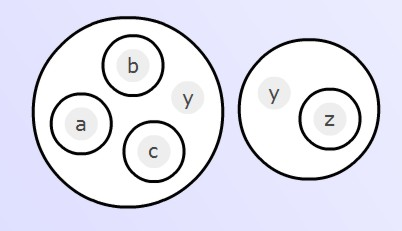The first step is to copy the second factor into the circle that contains the letter a: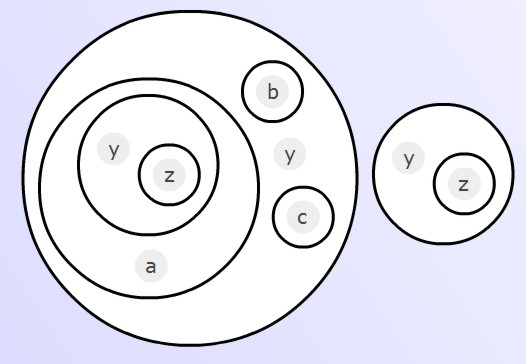The y in that first inner factor can be uncopied, then the z unwrapped, to give us: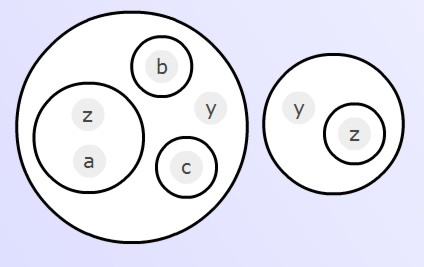Now, exactly the same operation may be performed on the remaining inner factors, not including the factor y, giving us: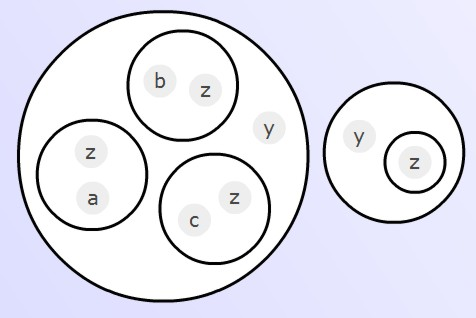Our final task is to get rid of the right-hand factor. This is done (as in the proof of distribute) by copying the left-hand factor into it:The y in the copied factor may be uncopied; while (z) may be copied into each inner factor, giving us: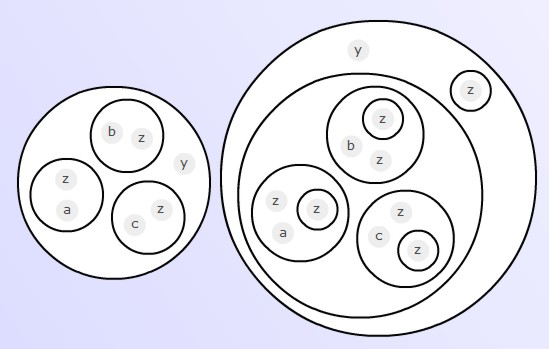But each z (z) = z O = O is equivalent to an empty circle, which causes each of the inner factors to vanish, leaving us with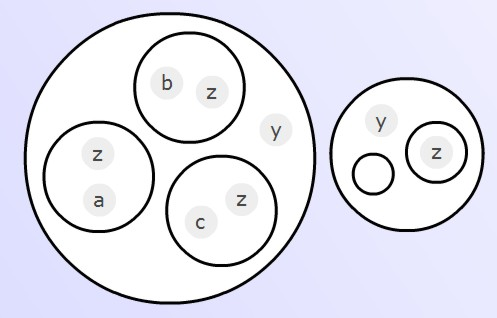Then y and (z) are destroyed by the empty circle, causing the second factor to vanish altogether. Therefore the original expression is equivalent to: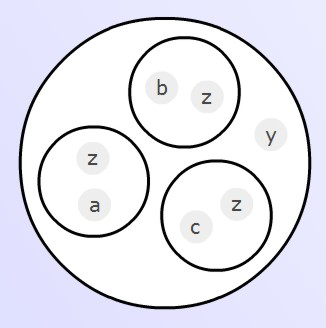- quod erat demonstrandum.

We have demonstrated the result with three inner factors, but it is clear that the same proof would work, however many inner factors there were. The reverse of modified distribute is of course modified collect. The importance of this reverse operation is that it collects up several instances of the letter z, even in the presence of the expression y, which is not necessarily of the form (ez).

Here is an instance of the CL-Calculus Play Area. Please use it to run through the above series of steps!

2. reduce-depth

We prove the reverse transformation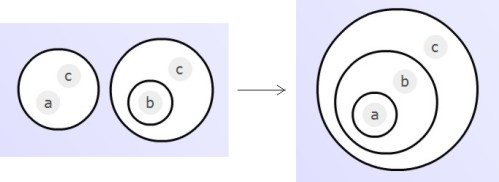Starting from the expression on the left, the steps are 1) copy the first factor into the circle with the letter b: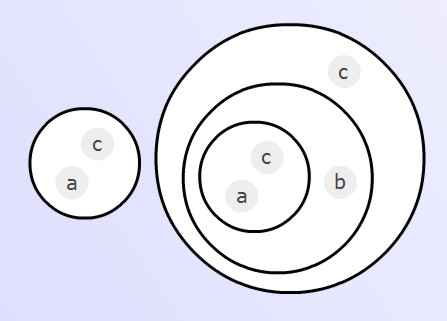2) uncopy the letter c: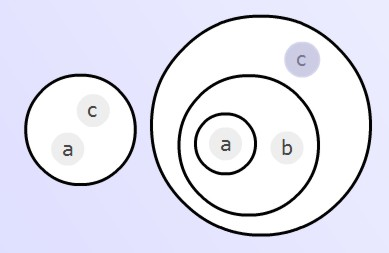3) Get rid of the first factor by copying the second factor into it:4) Uncopy a and c from the copied factor. It will be seen that this causes the whole of the first factor to vanish, leaving us with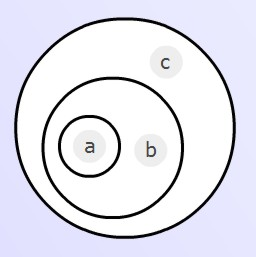as required.

The operation of depth reduction may be extended to the case where there are several factors, rather than just one, at depth 2: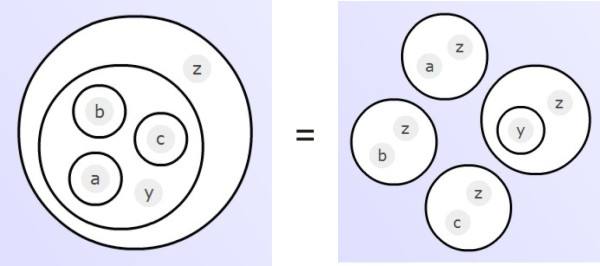Again, we prove this in reverse, starting from the right-hand side of the equation. Copy all the factors (az), (bz), (cz) etc. into the circle with y; then uncopy all the z's out of these factors: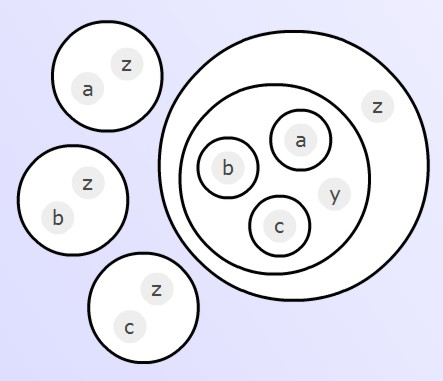We now get rid of all the factors except the last by copying the last factor into them in turn. For example, the first factor, (az), becomes: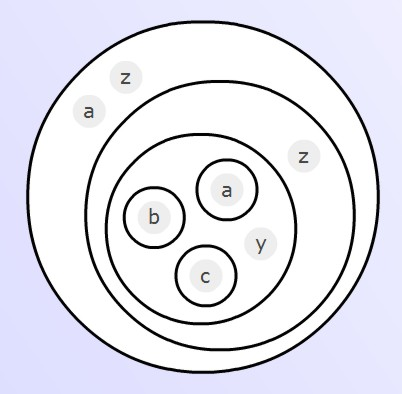Uncopying the z and the a, we see that this factor quickly reduces to nothing. The same applies to the other factors (bz), (cz) etc., leaving us with just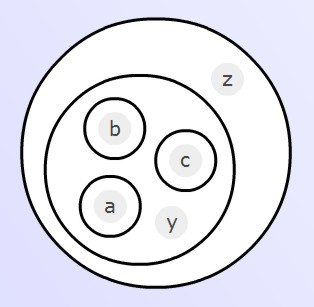Q.E.D.

3. flip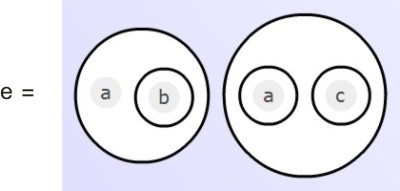Note the characteristic feature of this expression. If _ is substituted for a, then e becomes b; if a=O, then e=c. The point of flip is that it converts e into an alternative (prime) form which is sometimes more convenient. The first move is to copy the first factor into the circle that contains just the letter a: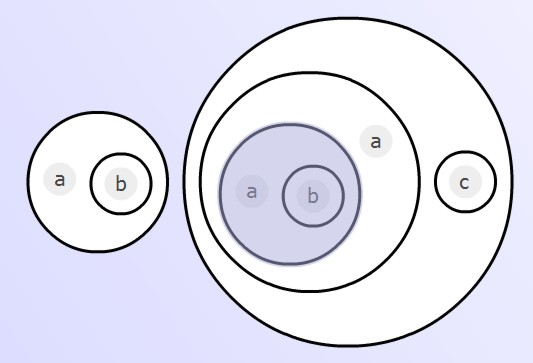This quickly turns into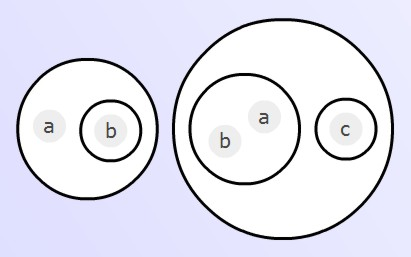Now copy the first factor into the circle containing just c: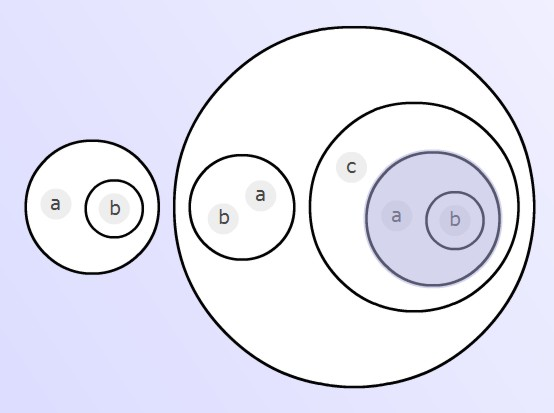then copy the a at depth 3 into the circle containing b at depth 4: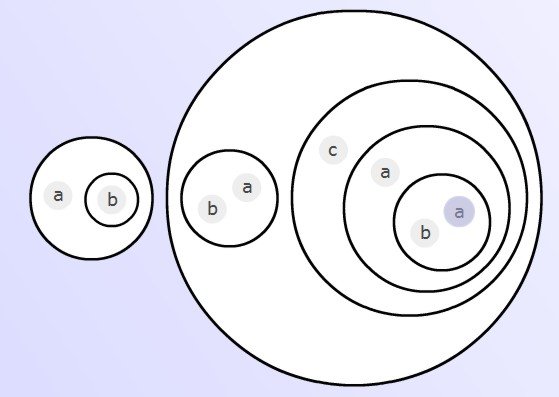then the expression (ab) may be uncopied, leaving us with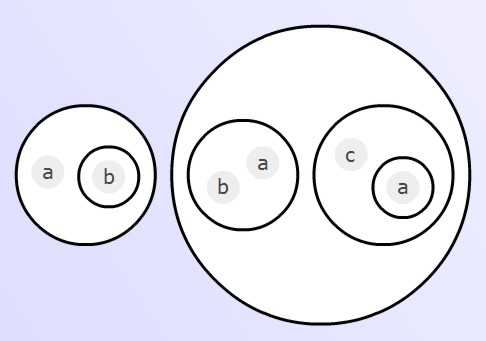Finally, get rid of the first factor in the usual way, by copying the second into it:Uncopy first the two instances of the letter a, then (b). The first factor quickly reduces to nothing. Thus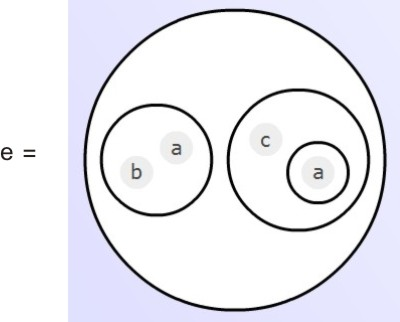Again, if a=_ then e=b; but if a=O then e=c. It's just another way of putting it.

4. each way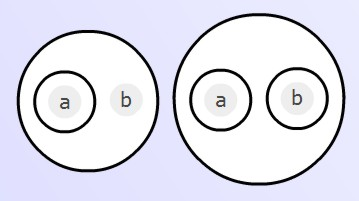If b=_, the first factor reduces to a, the second vanishes; if b=O, the first factor vanishes, the second reduces to a. Either way, the expression reduces to a - hence the name each way. But note that this is a mere observation: it has nothing to do with proving that the expression is equivalent in the CL-calculus to the expression a. To do that, we must proceed in the usual way, starting by copying the left-hand factor into the circle which contains just the letter b: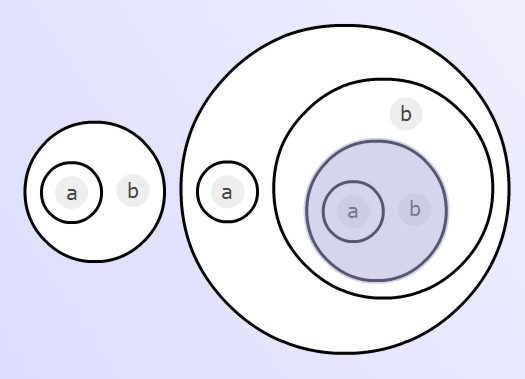By uncopying the b and the (a) from depth 3, we convert the right-hand factor into an expression that easily reduces to a. The expression as a whole is now: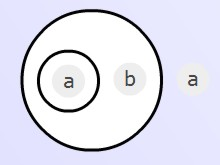Now uncopy the a from depth 2: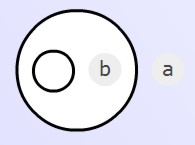which reduces to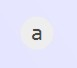Here are some proofs of the same equations in a different style, all making use of the already proven operation distribute, and some making use of complements:

1. modified distribution

(y(z)) ( (a) (b) (c) ... y) = (y(z)) ( (a) (b) (c) ... ((y)) )   [enfold]
= ( (a(y(z))) (b(y(z))) (c(y(z))) ... ((y)(y(z))) )   [distribute]

For the last of the inner factors we have

((y)(y(z))) = ( (y) ( (y) y (z) ) )   [copy]
( (y) ( ( ) y (z) ) )   [uncopy]
= ( (y) (O) )   [destroy]
= y.

This factor y can then be used to uncopy the y's out of the other inner factors, giving us for the whole expression:

( (a(y(z))) (b(y(z))) (c(y(z))) ... ((y)(y(z))) ) = ( (a(y(z))) (b(y(z))) (c(y(z))) ... y )
= ( (az) (bz) (cz) ... y )   [uncopy, unwrap]

2. depth-reduction

(((a)b)c) = (( (a) ((b)) ) c)   [enfold]
= (( (ac) ( (b) c ) ))   [distribute]
= (ac) ( (b) c )   [unwrap]

The rule extends to as many inner factors as are required:

(( (a) (b) (c) ... y )z) = (( (a) (b) (c) ... ((y)) )z)   [enfold]
= (( (az) (bz) (cz) ... ((y)z) ))   [distribute]
= (az) (bz) (cz) ... ((y)z)   [unwrap]

3. flip

( a (b) ) ( (a) (c) ) = ( ( a (a(b)) ) ( c (a(b)) ) )   [distribute]
= ( (ab) ( c (a(b)) ) )   [uncopy]
= ( (ab) ( c (a(b(ab))) ) )   [copy]
= ( (ab) ( c (a(b(b))) ) )   [uncopy]
= ( (ab) ( c (a) ) )   [complements]

4. each way

a = ((a))
= ( (a) ( (b) ((b)) ) )   [complements]
= (( ( (a) b ) ( (a) (b) ) ))   [distribute]
= ( (a) b ) ( (a) (b) )   [unwrap]